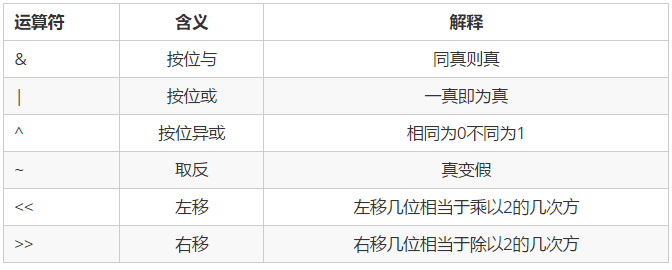0%

### 什么是位运算？## 奇偶校验:

num&1 奇数返回1 偶数返回0 0 返回0

~num + 1

<< 1, 2, 3, 4, 5

## 除 2, 4, 8, 16, 32

&#62;> 1, 2, 3, 4, 5

a = a^b
b = b^a
a = a^b

## 判断是否位2的幂次

1.N>0
2.N的二进制表示中只有一个1

def is_pow2(num):
return (n & (n-1) == 0)

## 消除x的最后一位的1

x & (x-1)

x = 1100
x-1 = 1011
x&(x-1) = 1000

C语言运算符优先级口诀：“单算移关与，异或逻条赋”。

• “单”表示单目运算符：逻辑非(!),按位取反(~),自增(++),自减(–),取地址(&),取值(&#42;)；
• “算”表示算术运算符：乘、除和求余(&#42;,/,%)级别高于加减(+,-)；
• “移”表示按位左移(<<)和位右移(&#62;>)
• “关”表示关系运算符：大小关系(>,>=,<,<=)级别高于相等不相等关系(==,!=)；
• “与”表示按位与(&)；
• “异”表示按位异或(^)；
• “或”表示按位或(|)；
• “逻”表示逻辑运算符：逻辑与(&&)级别高于逻辑或(||)；
• “条”表示条件运算符(? :)；
• “赋”表示赋值运算符(=,+=,-=,*=,/=,%=,>>=,<<=,&=,^=,|=,!=)；
• 逗号运算符(,) 级别最低